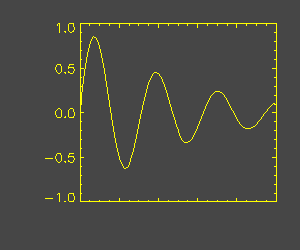# Turning Axis Labelling Off

QUESTION: I want to draw the axis, but I don't want the axis to be labelled. How can I turn axis labelling off?ANSWER: For an axis that is normally labelled with numbers, the easiest way to turn axis labelling off (e.g. the labelling for the X axis) is to use the XTICKFORMAT keyword set to the format "(A1)". For example, here is code that will produce a plot with the X axis labels turned off.

```   x = FINDGEN(100)
x = SIN(x/5) / EXP(x/50)
PLOT, x, XTICKFORMAT="(A1)"
```

Here is an example of how the axis looks with labelling turned off.[Return to IDL Programming Tips]